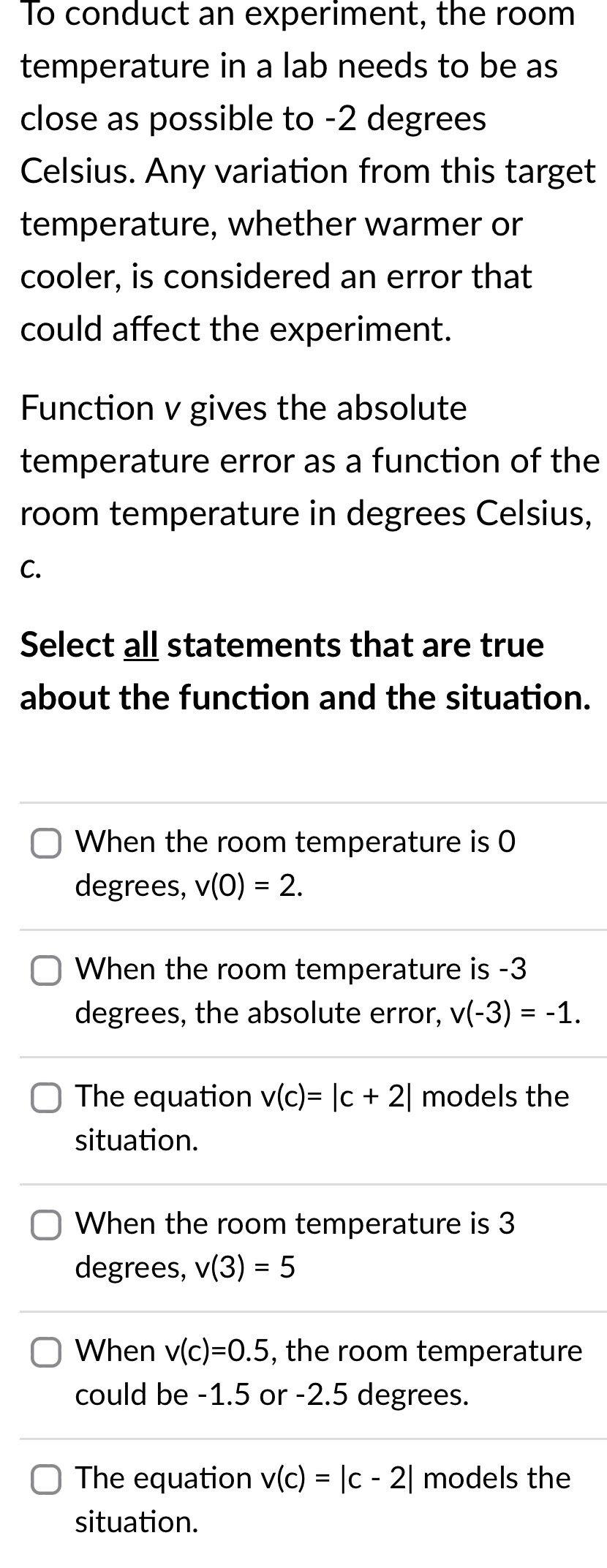### ¿Todavía tienes preguntas de matemáticas?

Pregunte a nuestros tutores expertos
Algebra
Pregunta

0To conduct an experiment, the room temperature in a lab needs to be as close as possible to $$- 2$$ degrees Celsius. Any variation from this target temperature, whether warmer or cooler, is considered an error that could affect the experiment. Function $$v$$ gives the absolute temperature error as a function of the room temperature in degrees Celsius, c. Select all statements that are true about the function and the situation. When the room temperature is $$0$$ degrees, $$v ( 0 ) = 2$$ . When the room temperature is $$- 3$$ degrees, the absolute error, $$v ( - 3 ) = - 1$$ . The equation $$v ( c ) = | c + 2 |$$ models the situation. When the room temperature is $$3$$ degrees, $$v ( 3 ) = 5$$

When $$v ( c ) = 0.5 ,$$ the room temperature could be $$- 1.5$$ or $$- 2.5$$ degrees. The equation $$v ( c ) = | c - 2 |$$ models the situation.